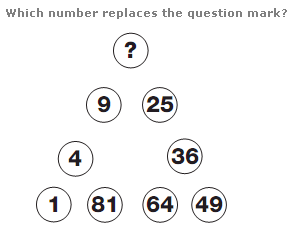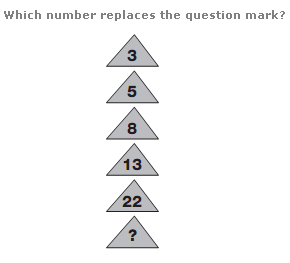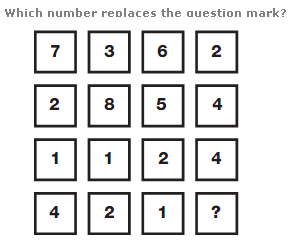# Puzzles - Number puzzles

### Exercise :: Number puzzlesAnswer : 16 Explanation : Starting bottom left and moving clockwise around the triangle, numbers follow the sequence of Square Numbers.Answer : 39 Explanation : Working from top to bottom, double each number and subtract 1, then 2, then 3 etc.Answer : 4 Explanation : Working in columns, the sum of the numbers in each column is always 14.